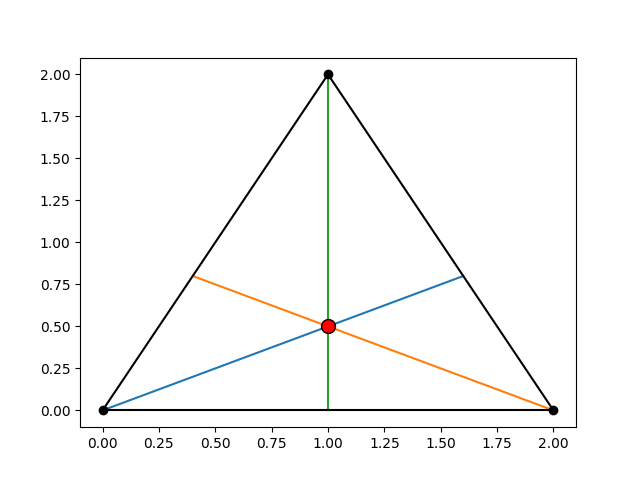# Triangle with Altitudes and Orthocenter¶

Plotting a triangle with its three altitudes and their intersection point, the orthocenter.Out:

```(<Figure size 640x480 with 1 Axes>, <AxesSubplot:>)
```

```from skspatial.objects import Triangle
from skspatial.plotting import plot_2d

triangle = Triangle([0, 0], [2, 0], [1, 2])

plot_2d(
triangle.plotter(c='k', zorder=3),
triangle.orthocenter().plotter(c='r', edgecolor='k', s=100, zorder=3),
*[x.plotter(c='k', zorder=3) for x in triangle.multiple('line', 'abc')],
*[x.plotter() for x in triangle.multiple('altitude', 'ABC')],
)
```

Total running time of the script: ( 0 minutes 0.149 seconds)

Gallery generated by Sphinx-Gallery